# Bear Put Spread Payoff, Break-Even and R/R

This page explains bear put spread profit and loss at expiration and the calculation of its maximum profit, maximum loss, break-even point and risk-reward ratio.

## Bear Put Spread Basic Characteristics

Bear put spread is a bearish strategy – it profits when underlying price goes down. The position consists of two put options (hence "put spread"):

• Buying a put with higher strike.
• Selling a put with lower strike and same expiration.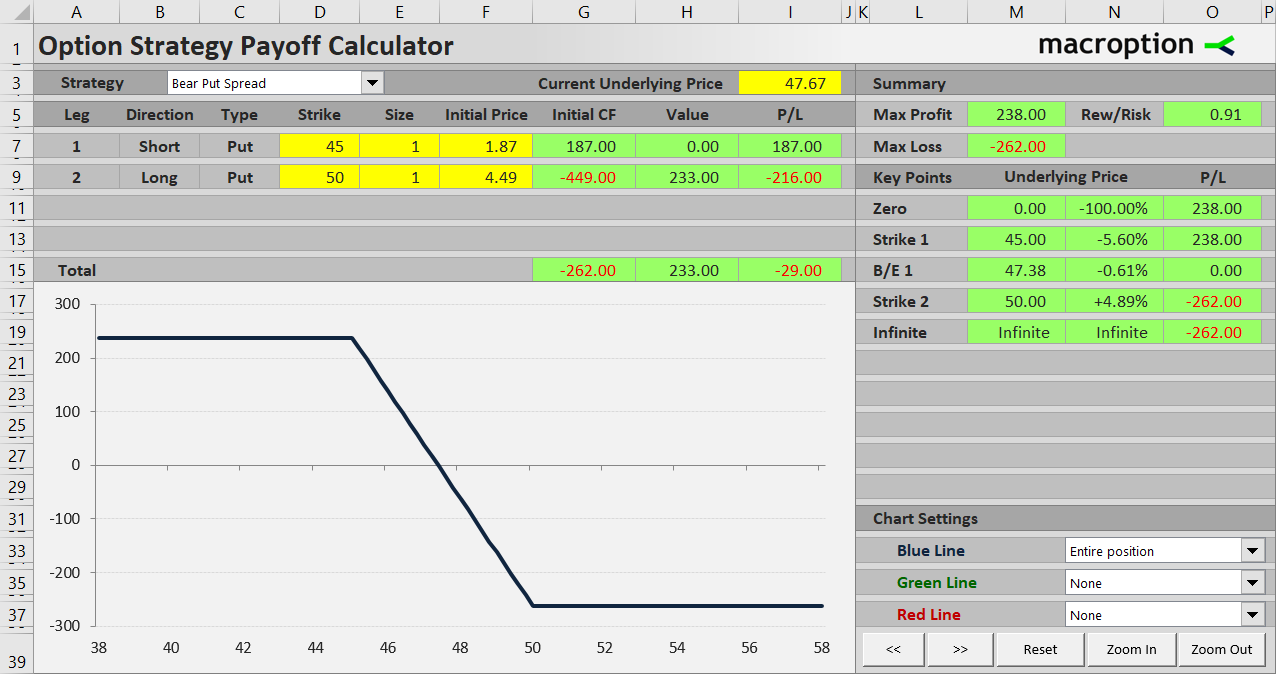An alternative name for this option strategy is long put spread, which might be a bit confusing. It does not refer to the underlying price exposure (which is bearish, so the word short would come to mind), but to the position in the put options themselves: you are long the higher strike put, which is typically more valuable and has a greater effect on your P/L than the short lower strike put – so you are basically "net long" put options. If you are still confused, see a more detailed explanation here.

Bear put spread is a debit spread, which means there is a net negative cash flow when opening the position. Again, the reason is that the higher strike put that you buy is more expensive than the lower strike put that you sell.

The trade has limited risk and limited profit potential. We will look at the payoff profile and the different scenarios below, using an example.

Let's set up a bear put spread using the following options:

• Buy one contract of a \$50 strike put option for \$4.49 per share, or \$449 total cash outflow.
• Sell one contract of a \$45 strike put with the same expiration for \$1.87 per share, or \$187 total cash inflow.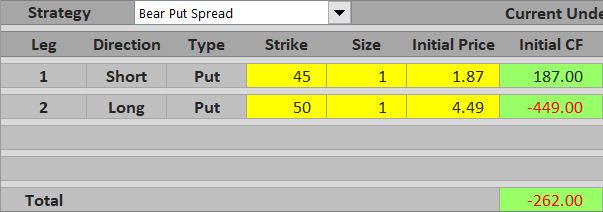Total cost of opening the position is \$449 – \$187 = \$262.

The idea behind the trade is that underlying price will go down and the difference between the two put options' values will increase, because the higher strike put is generally more sensitive to underlying price than the lower strike put, and both become more valuable when underlying price declines.

If you hold the options until expiration, there are three possible scenarios.

## Scenario 1 (Maximum Loss)

Let's start with the worst thing that can happen. When your expectations about the underlying price direction are wrong and the underlying ends up above both strikes (in our example above \$50), both put options will expire worthless. The value of the position at expiration will be zero. The total outcome of the trade will be equal to the initial cash flow, which in our example is negative \$262.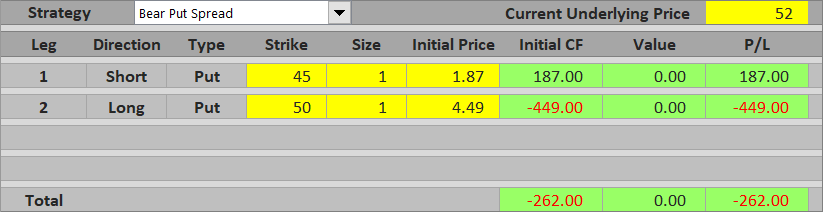Maximum loss from a bear put spread trade is equal to initial cost and applies when underlying price ends up at or below the higher strike.

The good thing is that the risk is limited. Regardless of how high the underlying will get at expiration, your loss is still the \$262 you paid in the beginning.

## Scenario 2 (Maximum Profit)

On the other end of the spectrum, if you are right about the underlying price direction and it ends up below the lower strike (for example, let's say \$42), both the put options will be in the money at expiration. You will exercise the higher strike put and gain \$8 per share (\$50 minus \$42), or \$800 for one contract. At the same time, you will be assigned the lower strike put and lose \$3 per share (\$45 minus \$42), or \$300. The combined result at expiration is \$500 = \$5 per share = the difference between the two strikes. With initial cost of \$262, your total profit from the trade is \$500 minus \$262 = \$238.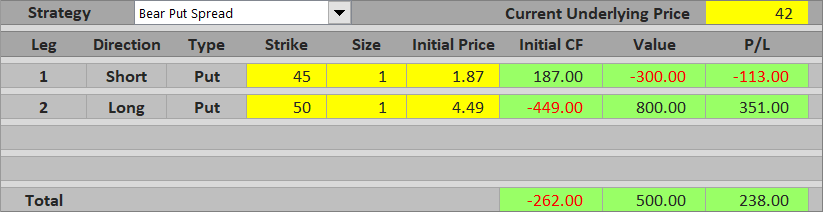Maximum possible profit from a bear put spread is the difference between the two strikes minus initial cost. It applies when the underlying ends up at or below the lower strike.

## Scenario 3 (Between the Strikes)

When the underlying price is between the two strikes at expiration, only the higher strike put will be in the money. The lower strike put is out of the money and expires worthless. The trade's total profit or loss depends on how far below the higher strike the underlying price gets and whether the amount you gain from exercising the higher strike put will be smaller or greater than the initial cost of the position.

For example, if the underlying ends up at \$47.67, you exercise the higher strike put and gain \$50 – \$47.67 = \$2.33 per share = \$233. This is less than what you initially paid for the spread and the trade's overall result is \$233 – \$262 = – \$29, a small loss.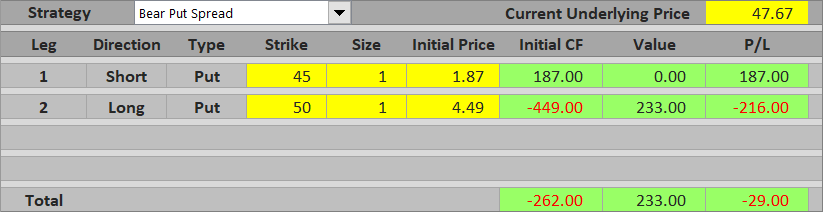In another example, if the underlying is \$46.50 at expiration, you will gain \$50 – \$46.50 = \$3.50 per share = \$350 from the higher strike put and the trade will make a net profit of \$350 – \$262 = \$88.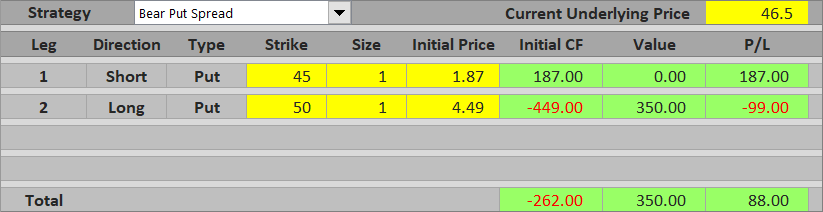In general, the payoff profile between the two strikes is linear and inversely related to underlying price. Near the lower strike, the overall P/L approaches maximum profit. Near the higher strike, it approaches maximum loss.

## Bear Put Spread Payoff Diagram

The chart below shows total profit or loss (blue) and contribution of the two options – the long higher strike put (red) and the short lower strike put (green).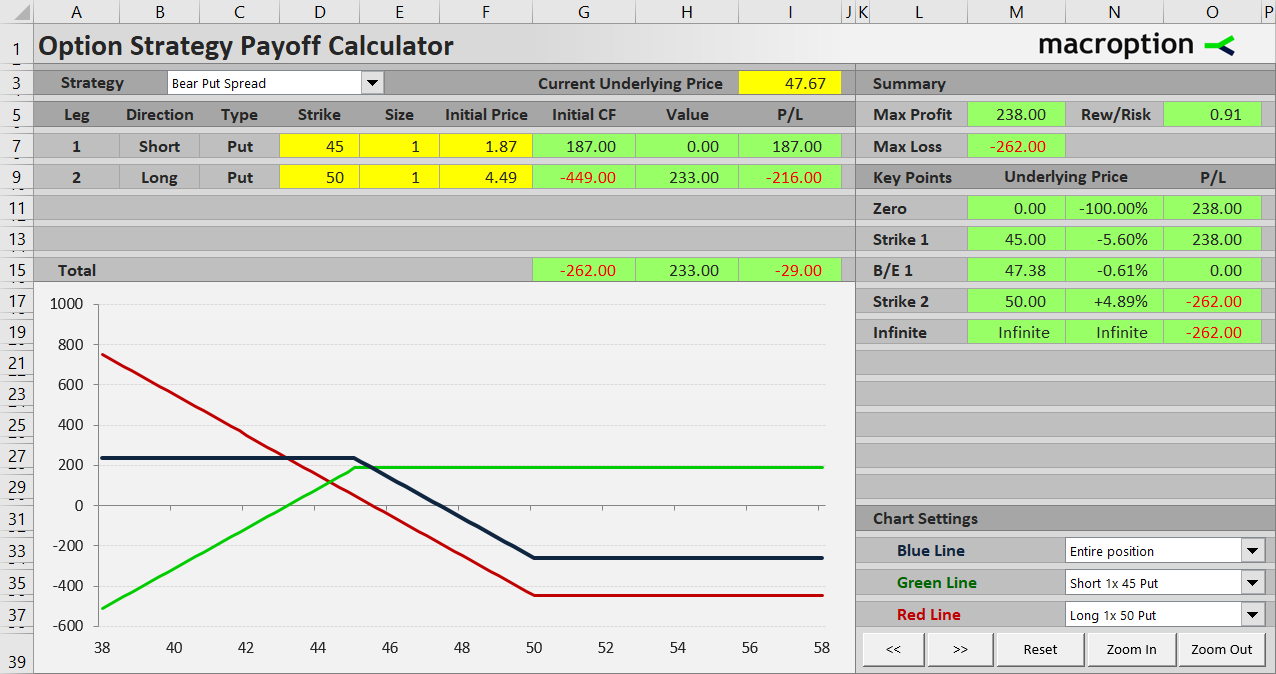Above \$50 both options are out of the money. Total loss is constant and equal to initial cost, or maximum risk of the trade.

Between \$45 and \$50 total P/L rises as underlying price declines and the higher strike put becomes more valuable. The lower strike put is still out of the money.

Below \$45 both the options are in the money and their values continue to rise as underlying price decreases. But their payoffs exactly offset one another and total P/L remains constant, equal to maximum possible profit.

## Bear Put Spread Break-Even Point

The break-even point – the exact point between the two strikes where the total P/L turns from loss to profit, or vice versa – is very easy to calculate. It is the underlying price that makes the higher strike put option's value exactly equal to the initial cost of the entire position.

In our example, initial cost is \$262 or \$2.62 per share. The underlying price at which the \$50 strike put is worth \$2.62 is \$50 minus \$2.62 = \$47.38.

The general formula for bear put spread break-even point is:

B/E = higher strike – initial cost

## Bear Put Spread Risk-Reward Ratio

Another useful statistic which gives you an idea about a potential trade's payoff profile is the risk-reward ratio, calculated simply as the ratio between maximum possible loss (risk) and maximum possible profit (reward).

We have already calculated the two for our example: maximum risk is \$262 (initial cost of the position, scenario 1) and maximum profit is \$238 (scenario 2).

Different people like to express the risk-reward ratio in different ways. If you like the "risk:reward" format, it is 262:238 or 1:0.91. I like to express it as a single number – reward as multiple of risk, which in our case is 0.91.

The general formulas for bear put spread risk and reward are the following:

Maximum loss (risk) = initial cost

Maximum profit (reward) = higher strike – lower strike – initial cost

... and also:

Maximum profit (reward) = B/E – lower strike

## Similar Option Strategies

Bear put spread payoff profile is similar to bear call spread, which uses call options instead of puts and it is a credit spread (net positive initial cash flow).

Bear put spread and bear call spread are inverse to bull put spread and bull call spread, respectively. The main difference is of course the opposite (bullish) exposure to underlying price.

Bear put spread is often considered a cheaper and less aggressive alternative to the plain and simple long put, which is effectively a bear put spread without the short lower strike put. The main advantages of bear put spread over long put are lower initial cost (because the lower strike put you sell helps pay for the more expensive higher strike put) and therefore higher break-even price and higher probability of profit. The main disadvantage is limited profit potential (maximum profit of a long put is also limited as the underlying can't drop below zero, but typically much higher than a spread's maximum profit).

A bear put spread's profit potential, break-even point and risk-reward ratio vary with selection of different strikes – if you choose a far out of the money strike for the short lower strike put, both initial cost and maximum potential profit will be higher and the payoff profile will be similar to a long put.

By remaining on this website or using its content, you confirm that you have read and agree with the Terms of Use Agreement.

We are not liable for any damages resulting from using this website. Any information may be inaccurate or incomplete. See full Limitation of Liability.

Content may include affiliate links, which means we may earn commission if you buy on the linked website. See full Affiliate and Referral Disclosure.

We use cookies and similar technology to improve user experience and analyze traffic. See full Cookie Policy.